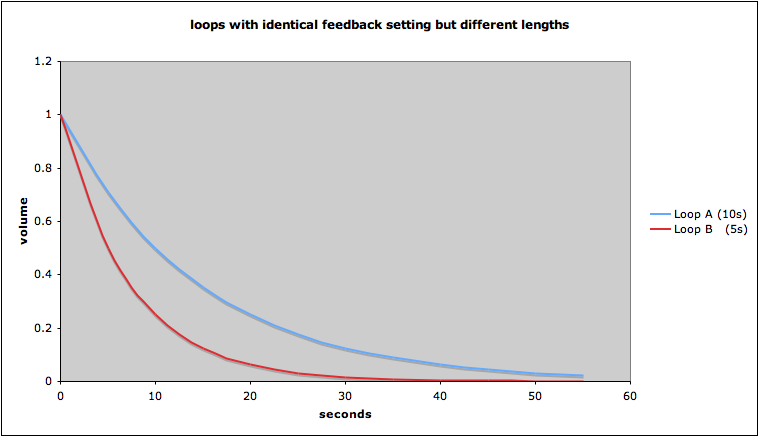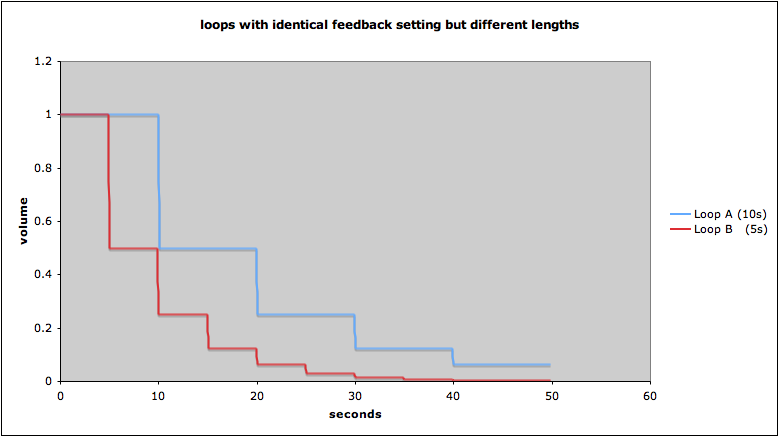# Loop Feedback Control

## Observation

The shorter a loop the more quickly it fades out.

## Explanation

The feedback control sets the amount by which the volume is reduced per loop repetition. Thus, a shorter loop's volume will decrease by the same amount within less time than a longer loop.

### Example

Feedback is set to 50%.
Loop A duration 10 s, feedback will decrease by 50% per repetition, i.e. every 10 seconds.
Loop B duration 5 s, feedback will decrease by 50% per repetition, i.e. every 5 seconds.
After 10 seconds Loop A will have diminished to 50%, Loop B will have diminished to 50% * 50% -> 25%(assuming the feedback was moved exponentially down from 100% to 50% during the first run of the loop)(assuming the feedback was moved briskly down from 100% to 50% during the first run of the loop. Thanks to Andy Butler for this graphic and for making the point.)

## Parameters

ParameterSymbolUnitRangeDomain
feedbackF% (percent)0-100integer
loop durationLDs (seconds)[0,...real
process durationPDs (seconds)[0,...real
repetitions (number of)R[0,...integer
volumeV??real
initial volumeV0??real

## Formulas

(1)V = V0 * (F/100)RResulting volume V at the end of repetition R for a loop with a feedback setting of F
(2)R = PD / LDNumber of repetitions depending on process duration and loop duration

## Examples

What volume factor x will a 10-second-loop have decreased to after 60 seconds with a feedback setting of 80% ?

V0 * x = V0 * (F/100)(PD/LD); (using formula (1))

x = (F/100)(PD/LD) = 80/10060/10 = 0.86 = 0.26;

What feedback setting F will lead to a decrease in Volume by 90% within 30 seconds for a 10 second loop?

V = V0 * ( 100 - 90 ) / 100; (decrease by 90%)

V0 * 0.1 = V0 * (F/100)(PD/LD); (using formula (1))

F = 0.1(1/3) * 100 = 46 (59 in MIDI terms).

How many repetions R does it take until a loop's volume will have decreased to 10% with a feedback setting of 90% ?

V = V0 * 0.1;

V0 * 0.1 = V0 * (F/100)R; (using formula (1))

0.1 = (9/10)R;

log (0.1) = R * log (0.9);

R = log(0.1) / log (0.9) = 22;

## Remarks

• Changes of the feedback setting will only be audible in the next repetition of the loop.
• Gestures with the feedback control will be recorded with the loop and become part of it.
• We're using 100 as the maximum for the feedback setting. When using the EDP, maximum setting is 127 (due to MIDI). Thus, the formula (1) above would read: V = V0 * (F/127)R
• Volume in the text refers to Amplitude (not Loudness).